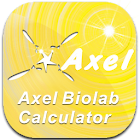Axel Biolab-Calculator

All Android applications categories

All Android games categories# Axel Biolab-Calculator

12 9.2

9.2 Users
rating

## Screenshots

Description

Axel biolab calculator is designed for:
Save time on searching and calculating for researchers, then strengthen its functions and add some relative documents. For instance, the origin of unit name is explained in the content of International System of Units in Knowledge Box; formulas for preparation of solutions are listed in Chemometrics. And other basic knowledge related to life science (i.e. Basic Laboratory Methods for Biotechnology, Statistics, Cell Culture, Signal Transduction and Common Drugs and Basic Instruments) is illustrated in a simple way to let beginners gain the basic concept of life science.

Partial Functions of Production of Dilution Solutions
1. Fill in the information of the solution about to produce (including molecular mass, concentration (touch the unit names to choose different units) and volume (touch the unit names to choose different units), and push the calculation bar to get the answer. And the same time, you could save the record.

2. When following the procedure mentioned above, in the meanwhile enter values for concentration and volume of dilution at the right side, to calculate the mass of stock solution needed, and the volume of desired target concentration after dilution.

3. Shift the bar of solution preparation to the right, and enter OD value and solution concentration to calculate the conversion of optical density OD260 (dsDNA、RNA、ssDNA and single-stranded oligonucleotide).

4. When entering the function of solution of dilution, touch the unit bar (the default value is OD (A260), and it can be shifted to OD260 and other units for different concentration calculation (M, g/ml and unit/ml) to calculate the needed solution for dilution.

Tags: biolab calculator , oligonucleotide od calculator

from 12 reviews

"Awesome"

9.2